## RD Sharma Class 9 Solutions Chapter 20 Surface Areas and Volume of A Right Circular Cone Ex 20.1

These Solutions are part of RD Sharma Class 9 Solutions. Here we have given RD Sharma Class 9 Solutions Chapter 20 Surface Areas and Volume of A Right Circular Cone Ex 20.1

Other Exercises

Question 1.
Find the curved surface area of a cone, if its slant height is 60 cm and the radius of its base is 21 cm.
Solution:
Radius of the base of the cone = 21 cm
and slant height (l) = 60 cmQuestion 2.
The radius of a cone is 5 cm and vertical height is 12 cm. Find the area of the curved surface.
Solution:
Radius of the base of a cone = 5 cm
Vertical height (h) = 12 cmQuestion 3.
The radius of a cone is 7 cm and area of curved surface is 176 cm2. Find the slant height.
Solution:
Curved surface area of a cone = 176 cm2
and radius (r) = 1 cm2Question 4.
The height of a cone is 21 cm. Find the area of the base if the slant height is 28 cm.
Solution:
Height of the cone (h) = 21 cm
Slant height (l) = 28 cm
∴ l2 = r2 + h2
⇒ r2 = l2– h2 = (28)2 – (21 )2
⇒ 784 – 441 = 343 …(i)
Now area of base = πr2
= $$\frac { 22 }{ 7 }$$ x 343 [From (i)]
= 22 x 49 = 1078 cm2

Question 5.
Find the total surface area of a right circular cone with radius 6 cm and height 8 cm.
Solution:
Radius of base of cone (r) = 6 cm
and height (h) = 8 cm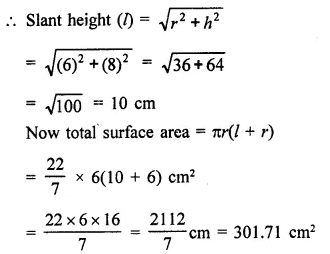Question 6.
Find the curved surface area of a cone with base radius 5.25 cm and slant height 10 cm. [NCERT]
Solution:
Radius of base of a cone (r) = 5.25 cm
and slant height (l) = 10 cm
Curved surface area = πrlQuestion 7.
Find the total surface area of a cone, if its slant height is 21 m and diameter of its base is 24 m. [NCERT]
Solution:
Slant height of a cone (l) = 21 m
and diameter of its base = 24 m
∴ Radius (r) = $$\frac { 24 }{ 2 }$$ = 12 m
Now total surface area = πr(l + r)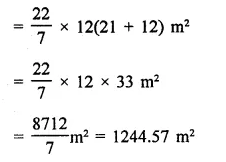Question 8.
The area of the curved surface of a cone is 60π cm2. If the slant height of the cone be 8 cm, find the radius of the base.
Solution:
Curved surface area of a cone = 6071 cm2
Slant height (l) = 8 cm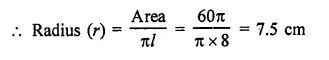Question 9.
The curved surface area of a cone is 4070 cm2 and its diameter is 70 cm. What is the slant height ? (Use π = 22/7).
Solution:
Surface area of a cone = 4070 cm2
Diameter of its base = 70 cmQuestion 10.
The radius and slant height of a cone are in the ratio of 4 : 7. If its curved surface area is 792 cm2, find its radius. (Use π = 22/7)
Solution:
Curved surface area of a cone = 792 cm2
Ratio in radius and slant height = 4:7
Then slant height = 7x
∴ Curved surface area πrl = 792Question 11.
A Joker’s cap is in the form of a right circular cone of base radius 7 cm and height 24 cm. Find the area of the sheet required to make 10 such caps. [NCERT]
Solution:
Radius of the base of a conical cap (r) = 7 cm
and height (h) = 24 cm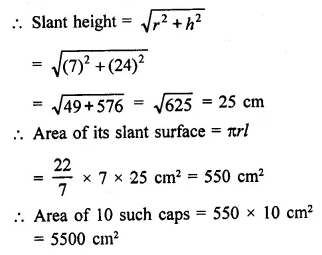Question 12.
Find the ratio of the curved surface areas of two cones if their diameters of the bases are equal and slant heights are in the ratio 4 : 3.
Solution:
Let diameters of each cone = d
Then radius (r) = $$\frac { d }{ 2 }$$
Ratio in their slant heights = 4 : 3
Let slant height of first cone = 4x
and height of second cone = 3x
Now curved surface area of the first cone = 2πrhQuestion 13.
There are two cones. The curved surface area of one is twice that of the other. The slant height of the later is twice that of the former. Find the ratio of their radii.
Solution:
In two cones, curved surface of the first cone = 2 x curved surface of the second cone
Slant height of the second cone = 2 x slant height of first cone
Let r1 and r2 be the radii of the two cones
and let height of the first cone = h
Then height of second cone = 2h
∴ Curved surface of the first cone = 2πr1h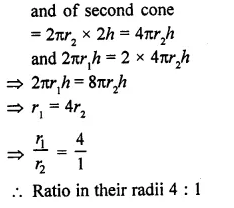Question 14.
The diameters of two cones are equal. If their slant heights are in the ratio 5 : 4, find the ratio of their curved surfaces.
Solution:
Let diameter of one cone = d
and diameter of second cone = d
∴ Radius of the first cone (r) = $$\frac { d }{ 2 }$$
and of second cone (r2) = $$\frac { d }{ 2 }$$
Ratio in their slant heights = 5:4
Let slant height of the first cone = 5x
Then that of second cone = 4x
Now curved surface of the first cone = 2πrh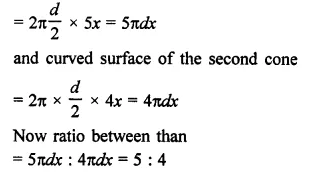Question 15.
Curved surface area of a cone is 308 cm2 and its slant height is 14 cm. Find the radius of the base and total surface area of the cone. [NCERT]
Solution:
Area of curved surface of a cone = 308 cm2
and slant height (l) = 14 cmQuestion 16.
The slant height and base diameter of a conical tomb are 25 m and 14 m respectively. Find the cost of white-washing its curved surface at the rate of Rs. 210 per 100 m2. [NCERT]
Solution:
Slant height of a cone (l) = 25 m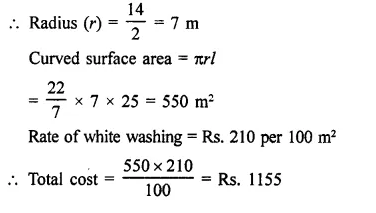Question 17.
A conical tent is 10 m high and the radius of its base is 24 m. Find the slant height of the tent. If the cost of 1 m2 canvas is Rs. 70, find the cost of the canvas required to make the tent. [NCERT]
Solution:
Height of conical tent (A) = 10 m
Radius of the base (r) = 24 m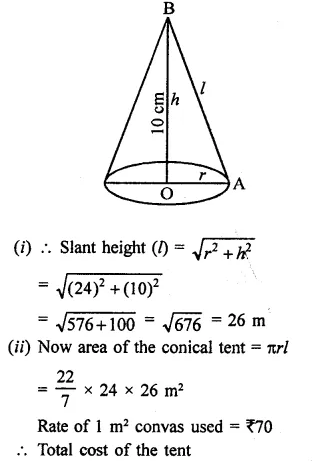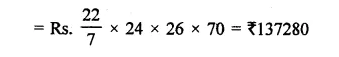Question 18.
The circumference of the base of a 10 m height conical tent is 44 metres. Calculate the length of canvas used in making the tent if width of canvas is 2 m. (Use π = 22/7)
Solution:
Circumference of the base of a conical tent = 44 mQuestion 19.
What length of tarpaulin 3 m wide will be required to make a conical tent of height 8 m and base radius 6 m? Assum that the extra length of material will be required for stitching margins and wastage in cutting is approximately 20 cm. (Use π = 3.14) [NCERT]
Solution:
Height of the conical tent (h) = 8 m
and radius of the base (r) = 6 mNow curved surface area of the tent = πrl = 3.14 x 6 x 10 = 188.4 m
Width of tarpaulin used = 3 m
∴ Length = 188.4 , 3 = 62.8 m
Extra length required = 20 cm = 0.2 m
∴ Total length of tarpaulin = 62.8 + 0.2 = 63 m

Question 20.
A bus stop is barricated from the remaining part of the road, by using 50 hollow cones made of recycled card-board. Each cone has a base diameter of 40 cm and height 1 m. If the outer side of each of the cones is to be painted and the cost of painting is ₹12 per m2, what will be the cost of painting these cones. (Use π = 3.14 and $$\sqrt { 1.04 }$$ = 1.02) [NCERT]
Solution:
Diameter of the base of tent = 40 cm
∴ Radius of the base of cone (r) = $$\frac { 40 }{ 2 }$$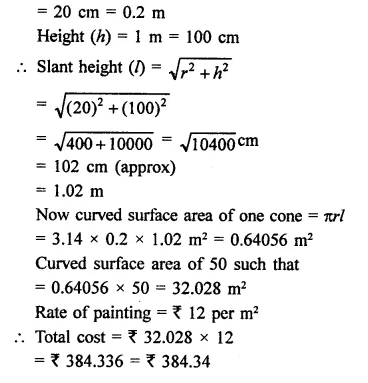Question 21.
A cylinder and a cone have equal radii of their bases and equal heights. If their curved surface areas are in the ratio 8 : 5, show that the radius of each is to the height of each as 3 : 4.
Solution:
Let radius of cylinder = r
and radius of cone = r
and let height of cylinder = h
and height of cone = h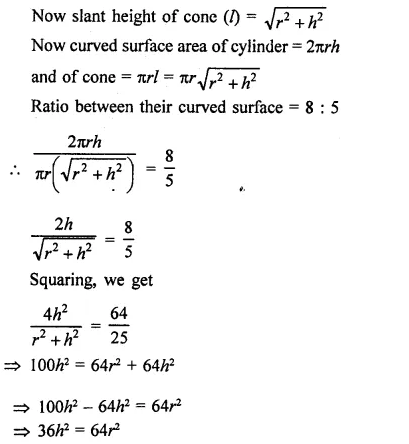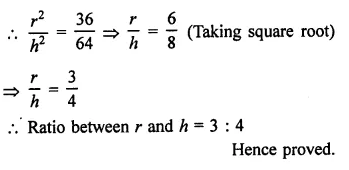Question 22.
A tent is in the form of a right circular cylinder surmounted by a cone. The diameter of cylinder is 24 m. The height of the cylindrical portion is 11 m while the vertex of the cone is 16 m above the ground. Find the area of the canvas required for the tent.
Solution:
Diameter of the cylindrical portion = 24 m 24
∴ Radius (r) = $$\frac { 24 }{ 2 }$$ = 12 m
Height of cylindrical portion = 11 m
and total height = 16 m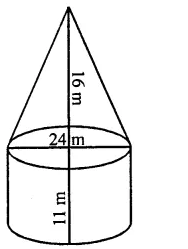∴ Height of conical portion = 16 – 11 = 5 m
∴ Slant height of the conical portion (l)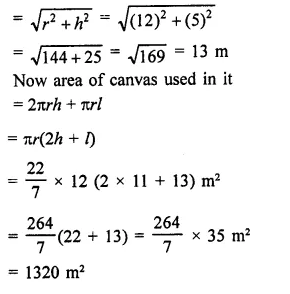Question 23.
A circus tent is cylindrical to a height of 3 meters and conical above it. If its diameter is 105 m and the slant height of the conical portion is 53 m, calculate the length of the canvas 5 m wide to make the required tent.
Solution:
Diameter of the cylindrical tent = 105 m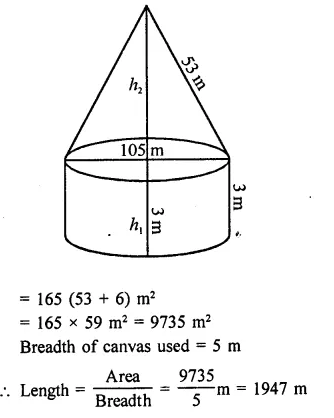Hope given RD Sharma Class 9 Solutions Chapter 20 Surface Areas and Volume of A Right Circular Cone Ex 20.1 are helpful to complete your math homework.

If you have any doubts, please comment below. Learn Insta try to provide online math tutoring for you.